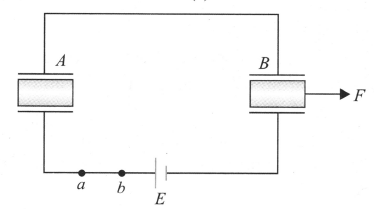Insertion of dielectric in capacitor
Question

# Two identical capacitors with identical dielectric slabs in between them are connected in series as shown in figure. Now, the slab of one capacitor is pulled out slowly with the help of an external force F at steady state as shown. Mark the correct statement(s).Moderate
Solution

## As the dielectric slab is pulled out, the equivalent capacity of the system decreases, and hence charge supplied by the battery decreases as potential of the system remains constant. It means charging of battery takes place and a positive charge flows from a to b. As the two capacitors are connected in series, so charge on both capacitors remains the same at all instants. From energy conservation law, ${U}_{i}+{W}_{ext}={U}_{f}+$work done on battery   $+\Delta H$As dielectric slab is attracted by the plates of capacitors, to pull it out, F has to perform some work, i.e.,  ${W}_{ext}\left(F\right)>0.$

Get Instant Solutions